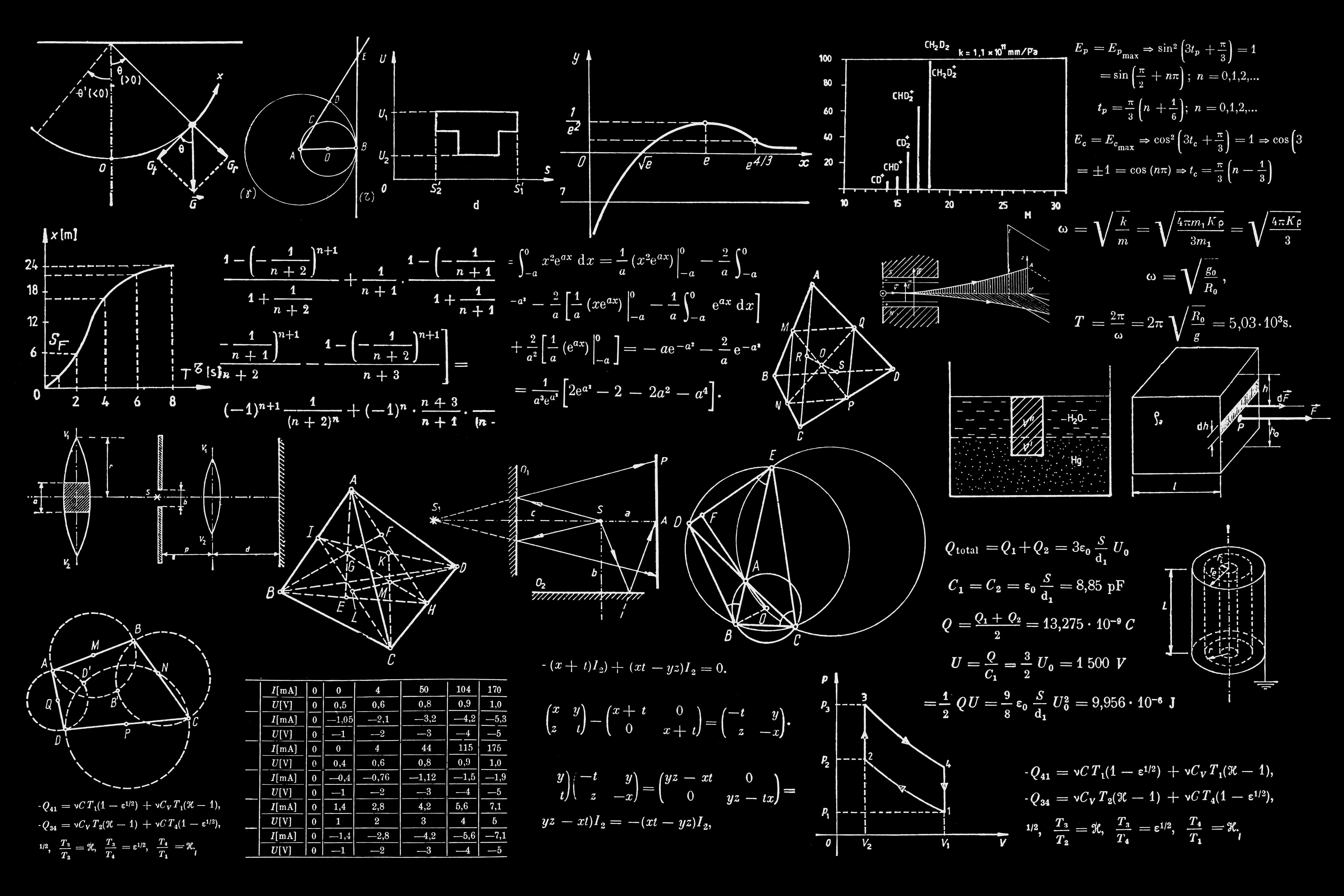# Mathematics for Machine Learning

A collection of resources to learn mathematics for machine learning.

## Mathematics for Machine Learning

by Marc Peter Deisenroth, A. Aldo Faisal, and Cheng Soon Ong

This is probably the place you want to start. Start slowly and work on some examples. Pay close attention to the notation and get comfortable with it.

## The Elements of Statistical Learning

by Jerome H. Friedman, Robert Tibshirani, and Trevor Hastie

Machine learning deals with data and in turn uncertainty which is what statistics aims to teach. Get comfortable with topics like estimators, statistical significance, etc.

If you are interested in an introduction to statistical learning, then you might want to check out “An Introduction to Statistical Learning”

## Probability Theory: The Logic of Science

by E. T. Jaynes

In machine learning, we are interested in building probabilistic models and thus you will come across concepts from probability theory like conditional probability and different probability distributions.

## Probabilistic Machine Learning: An Introduction

by Kevin Patrick Murphy

This book contains a comprehensive overview of classical machine learning methods and the principles explaining them.

## Multivariate Calculus by Imperial College London

by Dr. Sam Cooper & Dr. David Dye

Backpropagation is a key algorithm for training deep neural nets that rely on Calculus. Get familiar with concepts like chain rule, Jacobian, gradient descent,.

## Mathematics for Machine Learning – Linear Algebra

by Dr. Sam Cooper & Dr. David Dye

Agreat companion to the previous video lectures. Neural networks perform transformations on data and you need linear algebra to get better intuitions of how that is done.

## Mathematics for Deep Learning

This reference contains some mathematical concepts to help build a better understanding of deep learning.

## The Matrix Calculus You Need For Deep Learning

by Terence Parr & Jeremy Howard

In deep learning, you need to understand a bunch of fundamental matrix operations. If you want to dive deep into the math of matrix calculus this is your guide.

## Information Theory, Inference and Learning Algorithms

by David J. C. MacKay

When you are applying machine learning you are dealing with information processing which in essence relies on ideas from information theory such as entropy and KL Divergence,…

## Foundations of Machine Learning

by Mehryar Mohri, Afshin Rostamizadeh & Ameet Talwalkar

A comprehensive and accessible overview of the mathematics behind most learning algorithms (except deep learning). The appendix alone is worth a detour.

## Statistics and probability

A complete overview of statistics and probability required for machine learning.